# 十大经典排序算法¶

• 排序: 使一串记录，按照其中的某个或某些关键字的大小，递增或递减的排列起来的操作
• 稳定: 如果a原本在b前面，而a=b, 排序后a仍然在b前面
• 不稳定: 如果a原本在b前面，而a=b, 排序后a可能在b后面
• 内排序: 所有排序操作都在内存中完成
• 外排序: 由于数据太大放在磁盘中，排序通过内存和磁盘的传输才能进行
• 时间复杂度: 算法执行所耗费的时间
• 空间复杂度: 运行完一个程序所需内存的大小

# 各类排序算法的总结¶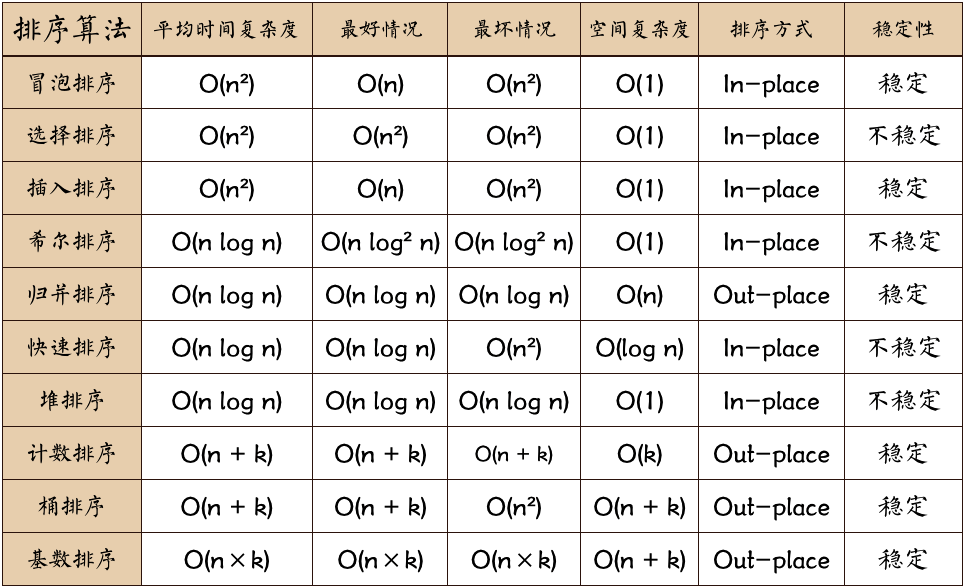• n: 数据规模
• k: "桶"的个数
• in-place : 排序过程占用常数内存，不申请额外辅助存储空间，输入数据在处理过程中会被改写，通常涉及交换和替换
• out-place: 排序过程需要申请不定量辅助存储空间进行计算和操作

# 分类¶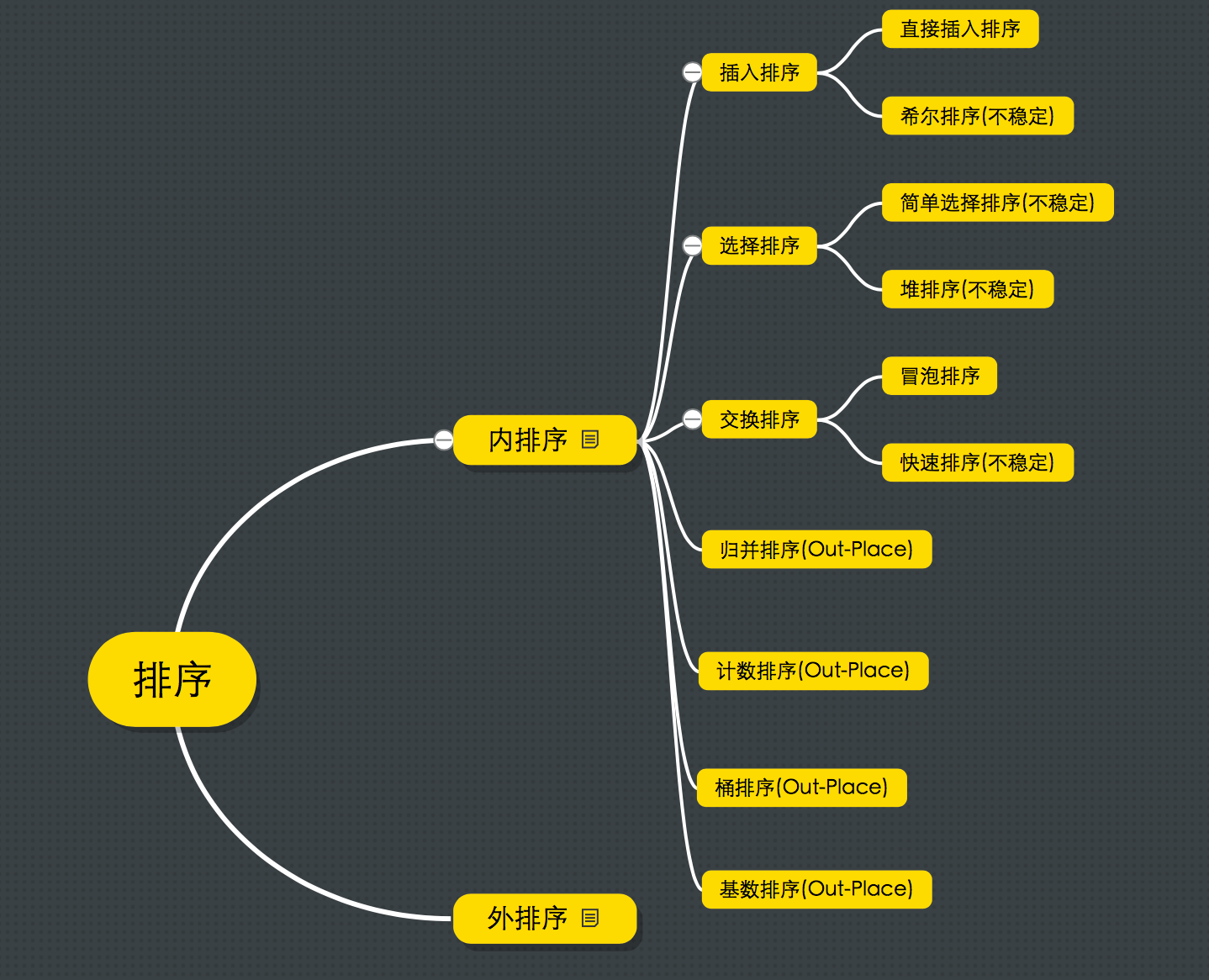# 下面是具体的各种算法及swift实现¶

## 冒泡排序¶

• 描述: 首先遍历整个序列，遍历过程中不断比较相邻两个元素，如果逆序就交换两元素的位置，一趟下来就把最大元素挪到了最后位置，然后把去掉最大元素的序列看成一个规模更小子序列再进行相同遍历操作，直到序列排序完成。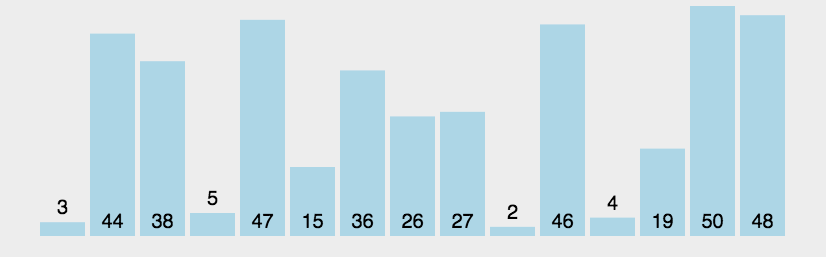import Foundation

func bubble_sort(_ array: inout [Int]) -> [Int] {

let count = array.count
for i in 0 ..< count - 1 {
for j in 0 ..< count-1-i {
if array[j] > array[j+1] {

let temp = array[j]
array[j] = array[j+1]
array[j+1] = temp
}
}
}
return array
}


## 简单选择排序¶

• 描述: 首先遍历序列，记录最小元素的下标或索引，遍历完成后，将最小元素和序列第一个元素进行位置交换，再将最小元素排除后序列看成一个规模更小的子序列重复之前遍历过程，直到整个序列有序。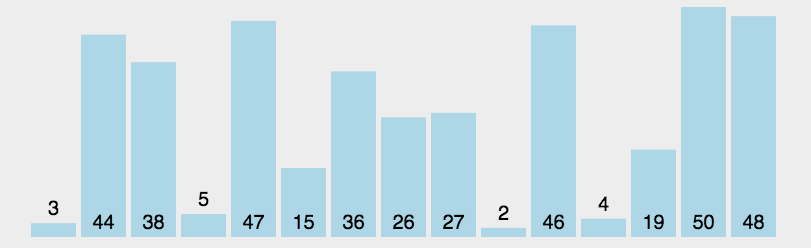import Foundation

func select_sort(_ array: inout [Int]) -> [Int] {

let count = array.count
for i in 0 ..< count-1 {
var minIndex = i
for j in (i+1) ..< count {
if array[j] < array[minIndex] {
minIndex = j
}
}
let temp = array[i]
array[i] = array[minIndex]
array[minIndex] = temp
}
return array
}


## 直接插入排序¶

• 描述: (类比于扑克牌整牌)首先把第一个元素看成是一个有序序列，把第二元素插入这个有序序列，插入过程从有序序列的尾部元素开始，逐个向前比较，如果插入元素大于或等于最后一个元素,就插入有序序列尾部，那么有序序列就扩大为2个元素，如果插入元素比最后一个元素小，继续向前查找比较，直到找到一个小于或者等于插入元素的元素，并将该元素后面所有包含在有序序列中的元素依次向后挪一位给插入元素空出位置(如果要插入的元素比有序序列中的所有元素都小，就把整个有序序列往后挪一个位置)，并放入所插入的元素，从而扩大有序序列的个数，如引循环，直到整个原始序列有序。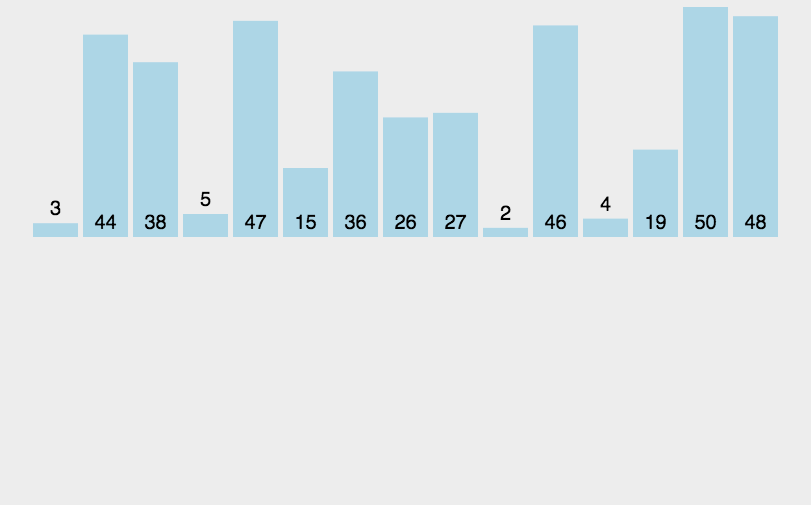import Foundation

func insert_sort(_ array: inout [Int]) -> [Int] {

let count = array.count

for i in 1 ..< count {
var insertIndex = i
for j in (0 ... i-1).reversed() {
if array[j] > array[i] {
array[j+1] = array[j]
insertIndex = j
}
}
array[insertIndex] = array[i]
}
return array
}


## 希尔排序¶

• 描述: 将整个待排序序列按增量序列多次分割成若干子序列进行直接插入排序，直到序列有序

#### 算法演示¶

• 索引增量(步长)为 5时，序列拆分为5个子序列，概念上如下图所示:
[83,               56               ]
[    1,                9            ]
[       67,               94        ]
[           0,                22    ]
[              84                 84]


[56,               83               ]
[    1,                9            ]
[       67,               94        ]
[           0,                22    ]
[              84                 84]




• 增量(步长)为 3时，再将上一步的结果序列拆分为3个子序列，如下:
[56,       0,      9,       84 ]
[   1,      84,     94         ]
[     67,    83,      22       ]


[0,       9,       56,      84 ]
[   1,      84,      94        ]
[     22,    67,      83       ]




• 增量(步长)为 1时，再将上一步的结果序列拆分为1个子序列，如下:

[0，1，9，22，56，67，83，84，84，94]




import Foundation

func shell_sort(_ array: inout [Int]) -> [Int] {

let count = array.count

var gaps = 
while(gaps.last! < count / 3) {
gaps.append(gaps.last! * 3)
}

for gap in gaps.reversed() {
for i in 0 ..< gap {
for j in stride(from: i + gap, to: count, by: gap) {
var insertIndex = j
let temp = array[j]
while(insertIndex>=gap &&  temp < array[insertIndex - gap]){
array[insertIndex] = array[insertIndex - gap]
insertIndex = insertIndex - gap
}
array[insertIndex] = temp
}
}
}

return array
}


#### 为什么希尔排序是不稳定的?¶

$[3^1 ,\quad , 3^3 , \quad]$ $[\,\quad ,3^2 , \quad , 1 ]$

$[3^1 ,\quad,3^3,\quad]$ $[\,\quad,1 ,\quad,3^2]$

## 归并排序¶

• 描述: 把待排序序列分成两个长度为$$n/2$$的子序列，对两个子序列分别进行归并排序(用到递归的思想)，再将两个有序的子序列合并成最终的整体有序序列，关键是归并操作的实现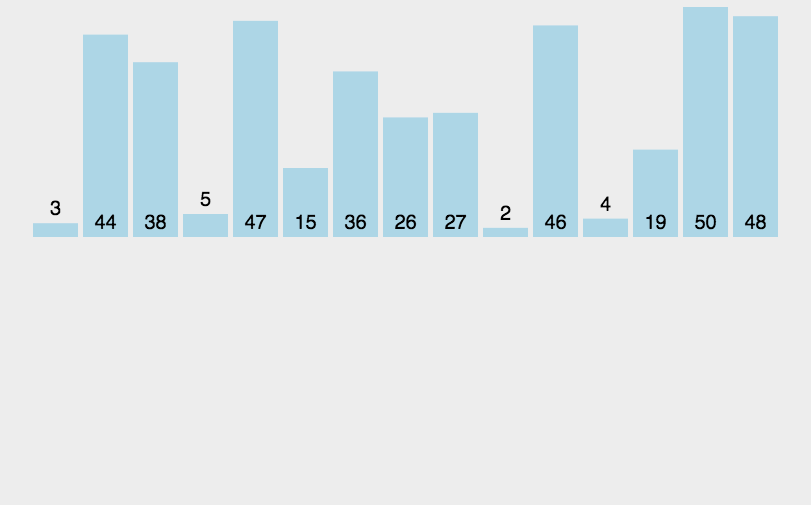import Foundation

func merge_sort(_ array: inout [Int]) -> [Int] {

let count = array.count

guard count >= 2 else {
return array
}

let mid = count / 2
var left =  Array(array[0 ..< mid])
var right = Array(array[mid ..< count])

return merge(left: merge_sort(&left), right: merge_sort(&right))
}

func merge(left: [Int], right: [Int]) -> [Int] {

var array = [Int]()

var leftIndex = 0;
var rightIndex = 0;

while leftIndex < left.count && rightIndex < right.count {

if left[leftIndex] < right[rightIndex] {
array.append(left[leftIndex])
leftIndex = leftIndex + 1
} else {
array.append(right[rightIndex])
rightIndex = rightIndex + 1
}

}

while leftIndex < left.count {
array.append(left[leftIndex])
leftIndex = leftIndex + 1
}

while rightIndex < right.count {
array.append(right[rightIndex])
rightIndex = rightIndex + 1
}

return array
}


## 快速排序¶

• 描述: 从待排序序列中挑一个元素作为基准值，将序列中所有比基准值小的元素放在前半部分，大于或等于基准值的放在后半部分，基准值放在中间，这个过程叫作以基准值对序列进行分区，分区后，前后两部份子序列再分别进行快速排序，递归的完成排序任务。排序的关键部分是分区函数的实现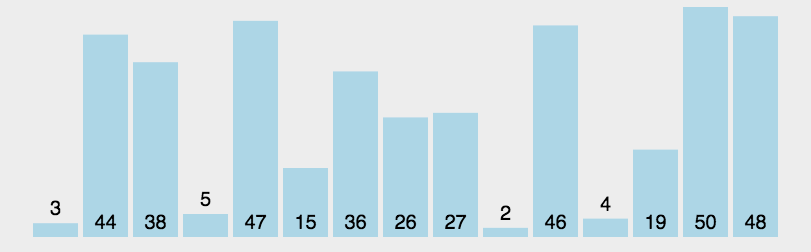import Foundation

func quick_sort(_ array: inout [Int]) -> [Int] {

quick_sort(&array, left: 0, right: array.count - 1)
return array
}

func quick_sort(_ array: inout [Int], left: Int, right: Int) {

if left < right {
let partionIndex = partition(&array, left: left, right: right)
quick_sort(&array, left: left, right: partionIndex - 1)
quick_sort(&array, left: partionIndex + 1, right: right)
}
}

func partition(_ array: inout [Int], left: Int, right:Int) -> Int {

let pivot = left

var index = pivot + 1

for i in index ... right {

if array[i] < array[pivot] {
array.swapAt(i, index)
index = index + 1
}
}

array.swapAt(left, index - 1)
return index - 1
}


## 堆排序¶

• 描述: 将初始待排序序列构建成大顶堆(顶部的元素是整个堆中最大的一个)，此堆为初始的无序区；将堆顶元素(即最大值)与最后一个元素交换，此时得到新的无序区(除最后一个元素之外的其它所有元素)和新的有序区(最后一个，也就是最大的那个元素)。由于交换后新的堆顶可能违反大顶堆的性质，因此需要对当前无序区重新调整为最大堆，然后再次将堆顶元素与无序区最后一个元素交换，得到新的无序区和新的有序区(最大的两个元素)。不断重复此过程直到无序区元素个数为1，则整个排序过程完成。

• 用数组模拟堆结构(完全二叉树结构):以下标0作为完全二叉树根结点，可以发现子结点的下标满足关系: $左子结点下标 = 2 * root + 1$ $右子结点下标 = 2 * root + 2$ 所以就可以通过数组下标来模拟二叉树结点操作，进行模拟出堆的相关操作，完全二叉树最后一个非叶子结点的下标: $floor(arr.count / 2) - 1$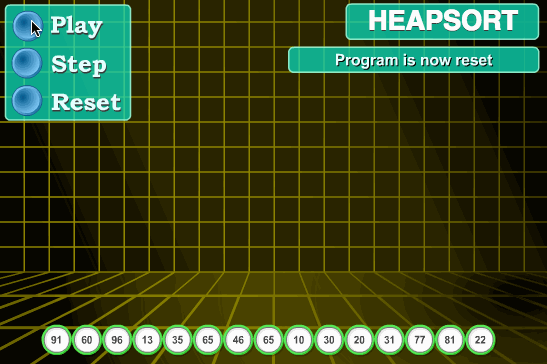import Foundation

func heap_sort(_ array: inout [Int]) -> [Int] {

buildMaxHeap(&array)

for i in (1 ..< array.count).reversed() {
array.swapAt(0, i)
heapify(&array, index: 0, length: i)
}

return array
}

func buildMaxHeap(_ array: inout [Int]) {

for i in (0 ..< array.count / 2).reversed() {
heapify(&array, index: i , length: array.count)
}

}

func heapify(_ array: inout [Int], index: Int, length: Int) {

let leftChild = index * 2 + 1;
let rightChild = index * 2 + 2;
var largest = index

if leftChild < length && array[largest] < array[leftChild] {
largest = leftChild
}

if rightChild < length && array[largest] < array[rightChild] {
largest = rightChild
}

if largest != index {
array.swapAt(largest, index)
heapify(&array, index: largest, length: length)
}
}


## 计数排序¶

• 描述: 获得序列的最大值、最小值，统计序列中每个元素出现的次数，存入数组，数组的下标对应序列元素的值，对所有计数累加，以累加值作为序列的下标索引，反向填充序列，完成排序任务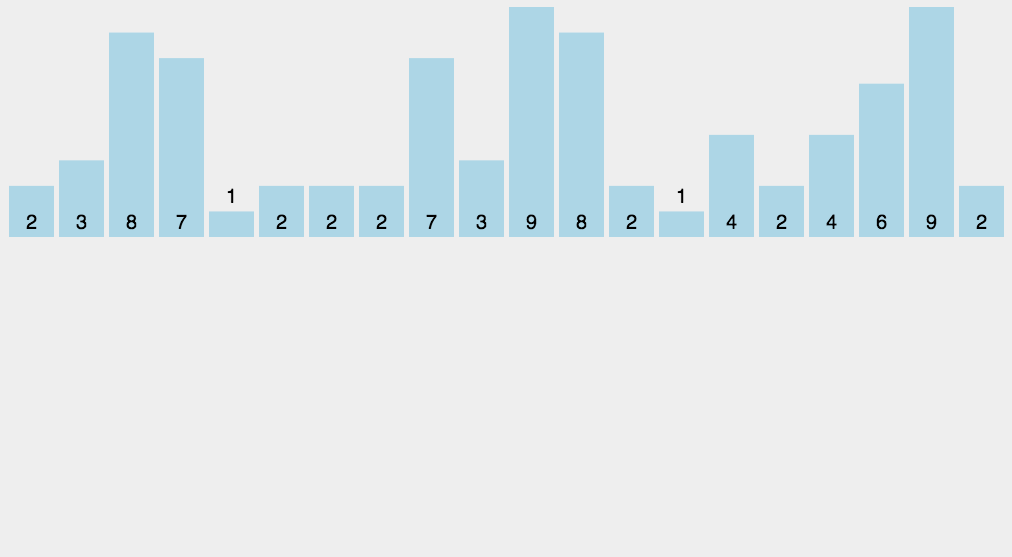import Foundation

func count_sort(_ array: inout [Int]) -> [Int] {

if let max = array.max() {

var bucket = Array(repeating: 0, count: max + 1)

for e in array {
bucket[e] = bucket[e] + 1
}

var index = 0
for k in 0 ... max {
while bucket[k] > 0 {

array[index] = k
index = index + 1

bucket[k] = bucket[k] - 1
}
}
}
return array
}


## 桶排序¶

• 描述: 在计数排序的基础上的改进版，先把序列分成若干桶，对每一桶可以采用之前的各种排序方法，对所有桶排序完成后，再把所有桶合并起来形成结果。由于用到了辅助存储桶，所以是Out-Place的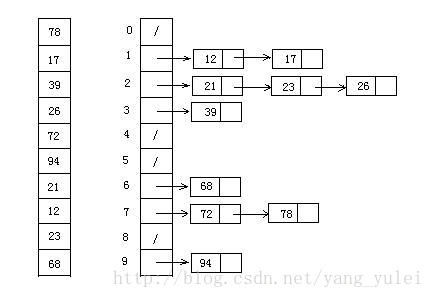import Foundation

func bucket_sort_test(_ array: inout [Int]) -> [Int] {
return bucket_sort(&array)
}

func bucket_sort(_ array: inout [Int], bucketSize: Int = 5) -> [Int] {

guard array.count > 0 else {
return array
}

let min = array.min()!
let max = array.max()!

let bucketCount = (max - min + 1) / bucketSize + 1
var bucket = Array(repeating: [Int](), count: bucketCount)

array.forEach { (e) in
bucket[(e - min + 1) / bucketSize].append(e)
}

var ret = [Int]()

for var b in bucket {
if b.count > 0 {
ret.append(contentsOf: count_sort(&b))
}
}

return ret
}


## 基数排序¶

• 描述: 基数排序是按照低位先排序，然后收集；再按照高位排序，然后再收集；依次类推，直到最高位。有时候有些属性是有优先级顺序的，先按低优先级排序，再按高优先级排序。最后的次序就是高优先级高的在前，高优先级相同的低优先级高的在前。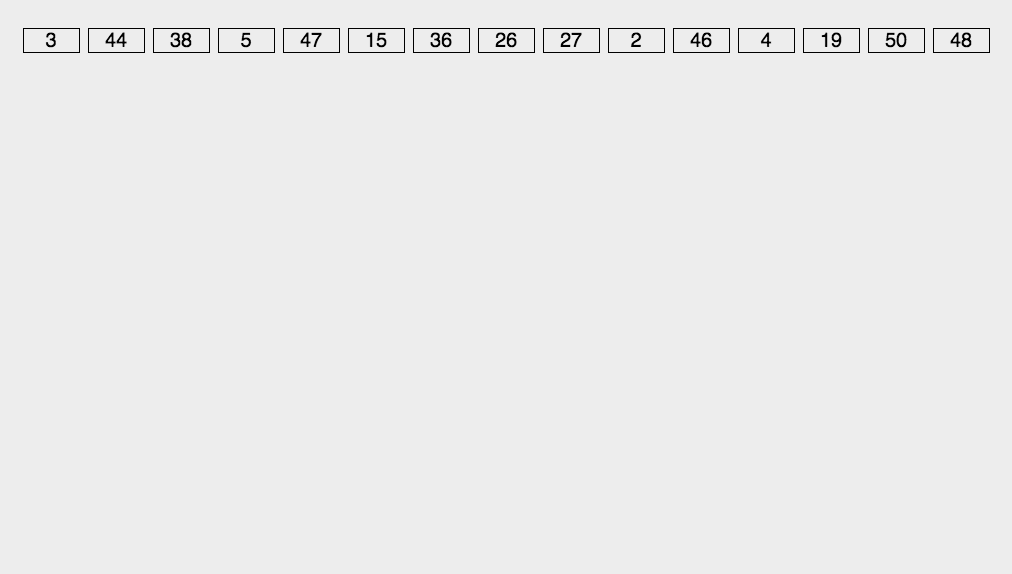import Foundation

func radix_sort_test(_ array: inout [Int]) -> [Int] {
}

func radix_sort(_ array: inout [Int], maxDigitCount: Int = 2) -> [Int] {

var dev = 1
for i in 0 ..< maxDigitCount {

var decimalBuckets = Array(repeating: [Int](), count: radix)

dev *= (i == 0) ? 1 : 10
for e in array {
let digit = (e / dev) % radix
decimalBuckets[digit].append(e)
}

var i = 0
decimalBuckets.forEach { (bucket) in
for e in bucket {
array[i] = e
i += 1
}
}
}

return array
}


# 总结¶

• 从规律上看，Out-Place类的排序都是稳定的排序
• 不稳定的排序只有：选择排序、希尔排序、快速排序和堆排序